2006-05-09 17:13:00 byxdaz 阅读数 7862

图像处理中常见变换

(1)KL变换
KL变换是遥感图像增强和信息提取中用得最多的线性变换，是对原波段图像进行波谱信息的线性投影变换，在尽可能不减少信息量的前提下，将原图像的高维多光谱空间的像元亮度值投影到新的低维空间，减少特征空间维数，达到数据压缩、提高信噪比、提取相关信息、降维处理和提取原图像特征信息的目的，并能有效地提取影像信息。它可使原来多波段图像经变换后提供出一组不相关的图像变量，最前面的主分量具有较大的方差，包含了原始影像的主要信息，所以要集中表达信息，突出图像的某些细部特征，可采用主分量变换来完成。

(2)去相关拉伸变换
通过去相关拉伸变换把相关性很高的波段进行去相关拉伸处理，减弱它们之间的相关性，然后进行拉伸，从而使深色区域的地物差异界线反映得更加清楚。

(3)纹理特征提取变换
纹理特征的提取方法比较简单，它是用一个活动的窗口在图像上连续滑动，分别计算出窗口中的方差、均值、最大值、最小值及二者之差和信息熵等，形成相应的纹理图像，当目标的光谱特性比较接近时，纹理特征对于区分目标可以起到积极的作用。选取适当的数据动态变化范围，进行纹理特征提取后，使影像的纹理特征得到突出，有利于提取构造信息。

(4)锐化增强
调整图像的锐化程度使地物在图像上的差别便于人眼识别，可达到信息增强的目的。对图像进行锐化增强实际上是利用变换函数把原图像进行灰度级转换，增大相邻像元的灰度值之差，从而达到突出图像细节的目的。

(5)定向滤波
利用定向滤波对ＴＭ图像频率特征进行筛选，将图像中的线与边缘特征信息增强，突出给定方向的线性影像信息，抑制其他方向的无用信息。采用45°方向滤波，滤波背景值用100%，滤波核设为3。滤波后突出了断裂的线性影像，断裂两侧的色调影像花纹明显不同，断裂造成的山脊错断等特征在影像上非常明显。

(6)缨帽变换
采用缨帽变换可以将ＴＭ图像除热红外波段的6个波段压缩成3个分量，其中的土壤亮度指数分量是6个波段的加权和，反映了总体的反射值；绿色植被指数分量反映了绿色生物量的特征；土壤特征分量反映了可见光和近红外与较长的红外的差值，它对土壤湿度和植物湿度最为敏感。这样的三个分量就是ＴＭ数据进行缨帽变换后的新空间，它可以对植被、土壤等地面景物作更为细致、准确的分析，应用这种处理方法可增强影像上深色区域的信息。

(7)芒塞尔彩色空间变换
在计算机内定量处理色彩时通常采用RGB（Red、Green、Blue）表色系统，但在视觉上定性的描述色彩时，采用HSV显色系统更直观些。Munsell HSV变换就是对标准处理彩色合成图像在红（R）、绿（G）、蓝（B）编码赋色方面的一种彩色图像增强方法，它是借助改变彩色合成过程中的光学参数的变化来扩展图像色调差异，将图像彩色坐标系中红、绿、蓝三原色组成的彩色空间(RGB)变换为由Hue(色度)，Saturation (饱和度)，value(纯度)三个变量构成的HSV色彩模型。其目的是为了更有效地抑制地形效应和增强岩石单元的波段差异，并通过彩色编码增强处理达到最佳的图像显示效果。HSV色彩模型能够准确、定量地描述颜色特征。

(8)非监督分类
遥感图像分类是将图像的所有像元按其性质分为若干个类别的技术过程，多光谱遥感图像分类是以每个像元的多光谱矢量数据为基础进行的，分类算法的核心是确定判别函数和相应的判别准则，如果我们事先没有类别的先验知识，在这种情况下对未知类别的样本进行分类的方法称之为非监督分类（Unsupervised Classification），非监督分类只能把样本区分为若干类别，而不能给出样本的描述。本次对中甸幅的小中甸盆地子区应用非监督分类中的K-均值算法，其基本思想是通过迭代，逐次移动各类的中心，直至得到最好的聚类结果为止，这种算法是一个迭代算法，迭代过程中类别中心按最小二程误差的原则进行移动，因此类别中心的移动是合理的。其缺点是要事先已知类别数，在实际中类别数通常根据实验的方法来确定。

(9）波段彩色合成
对两个波段的图像进行比值运算，可减弱背景而突出类别或目标信息，消除山影、云影等的影响，区分易混淆的地物。

(10)对数变换

(11)比值处理
比值处理采用高质量比值功能，使比值图像得到拉伸，有效地消除了地形影响，使阴影区的结构得到显示。处理出来的图像色彩丰富，既保留了原有地貌特征，又突出了线环构造，为盆地的研究提供了更为直观可靠的资料,立体感得到增强，阴影区结构清楚。

(12)小波变换

(13)付立叶变换

(14)HOUGH变换

(15)Karhunen-Loeve变换

(16)投影变换

2012-11-26 11:44:22 ivandark 阅读数 7108

KL变换是从n维特征中选取m维(m<n)，并使得选取出来的m维特征可以表示原来n维特征的信息（损失最小）。

Y = Φ X

|A - λE| = 0     解λ

An阶方矩，如果有数n维非零向量x，使得：

AX=x

则称：A的特征值；

xA对应于特征值的特征向量。

1.6Band图像

将6波段图像分波段读入内存。得到B1 ~ B6 六个图像矩阵

2.求所有波段图像的整体均值M（这里以整体散布为例，也可以进行类内散布类间散布，各有利弊）

3.求波段之间的协方差矩阵4.利用opencv函数 cvEigenVV();求特征值和特征向量

5.得到的特征向量可以按行拆分为第一 第二。。。。。第六主分量的6维特征向量

6.对每像素，用每维特征向量乘以中心化后的原像素灰度 累加  最终得到整幅变换后的图像（待定 不一定正确）

2017-11-15 16:28:30 u011779613 阅读数 861

PCA 方法是由 Turk 和 Pentlad 提出来的，它的基础就是 Karhunen-Loeve 变换(简称 KL变换)，是一种常用的正交变换。

PCA的变换矩阵是协方差矩阵，K-L变换的变换矩阵可以有很多种（二阶矩阵、协方差矩阵、总类内离散度矩阵等等）。当K-L变换矩阵为协方差矩阵时，等同于PCA。

2019-07-26 20:42:44 Arthur_Holmes 阅读数 558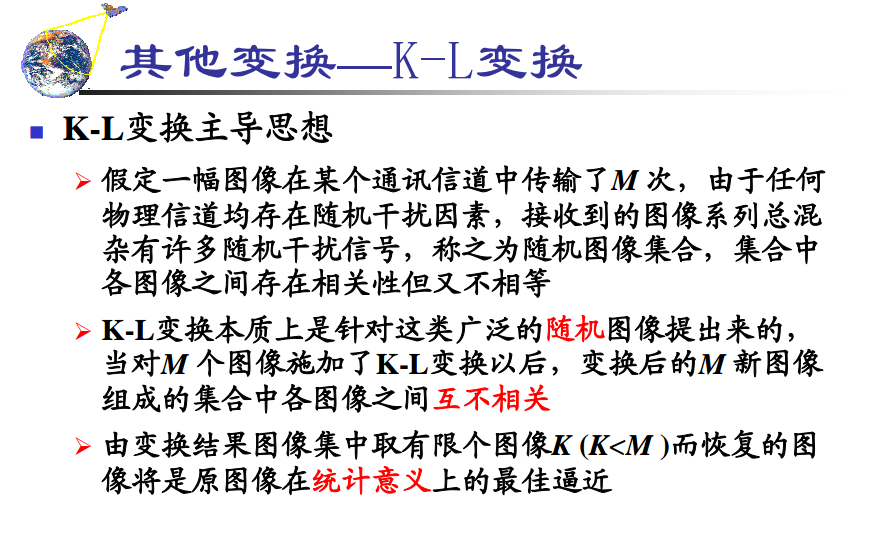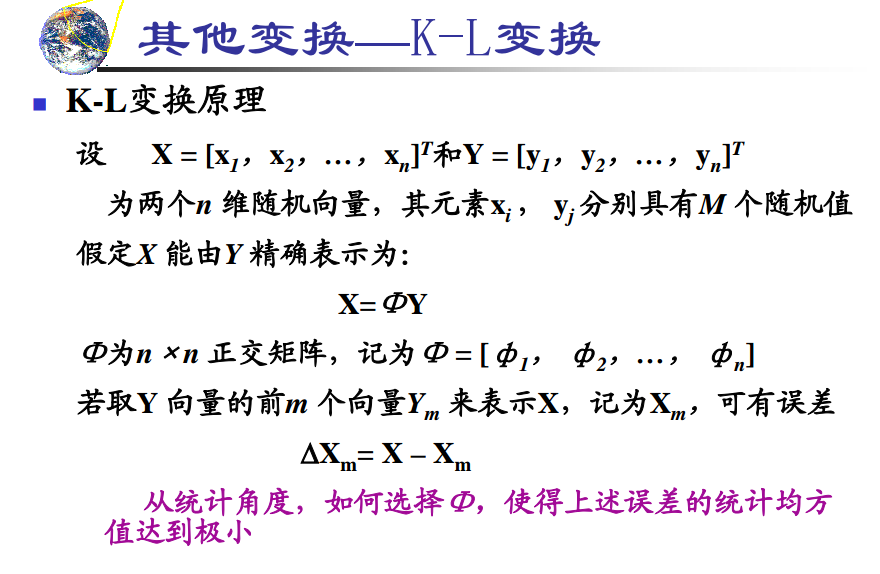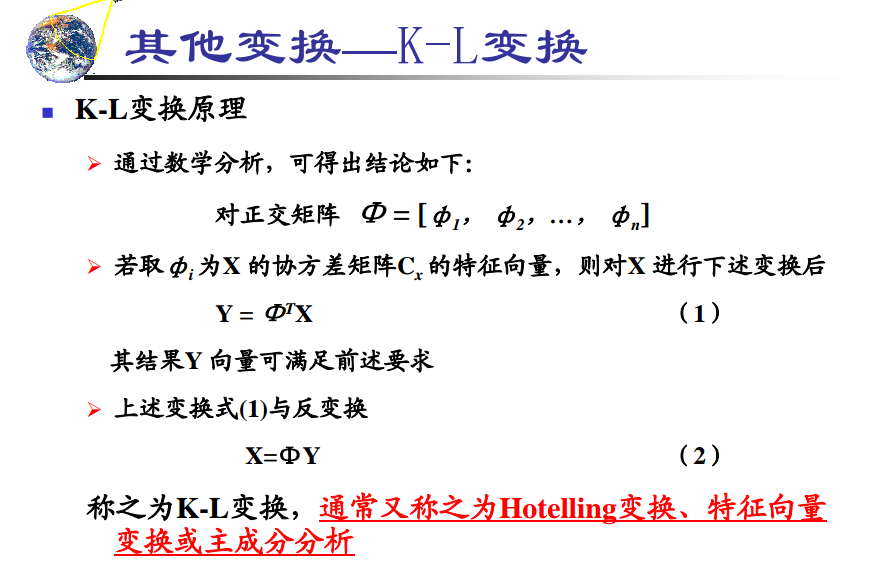https://zh.wikipedia.org/wiki/%E5%8D%8F%E6%96%B9%E5%B7%AE%E7%9F%A9%E9%98%B5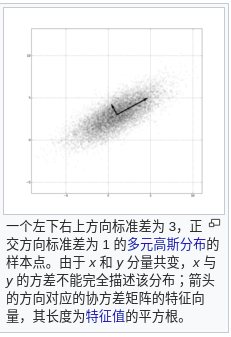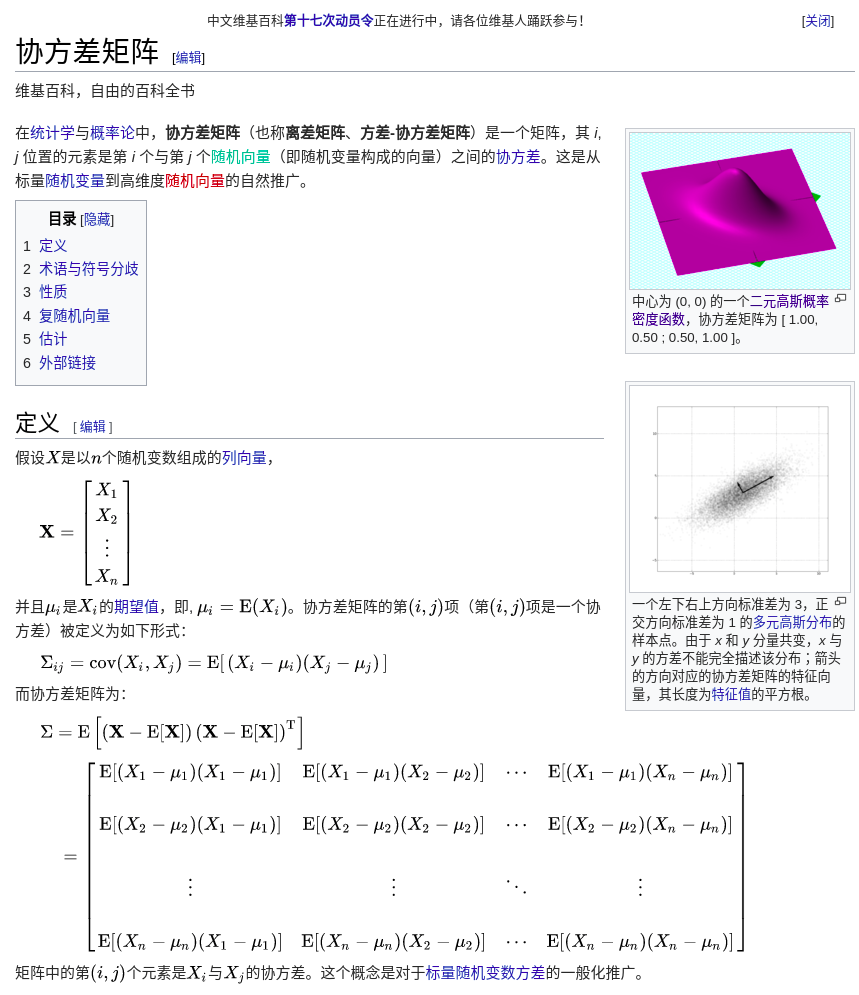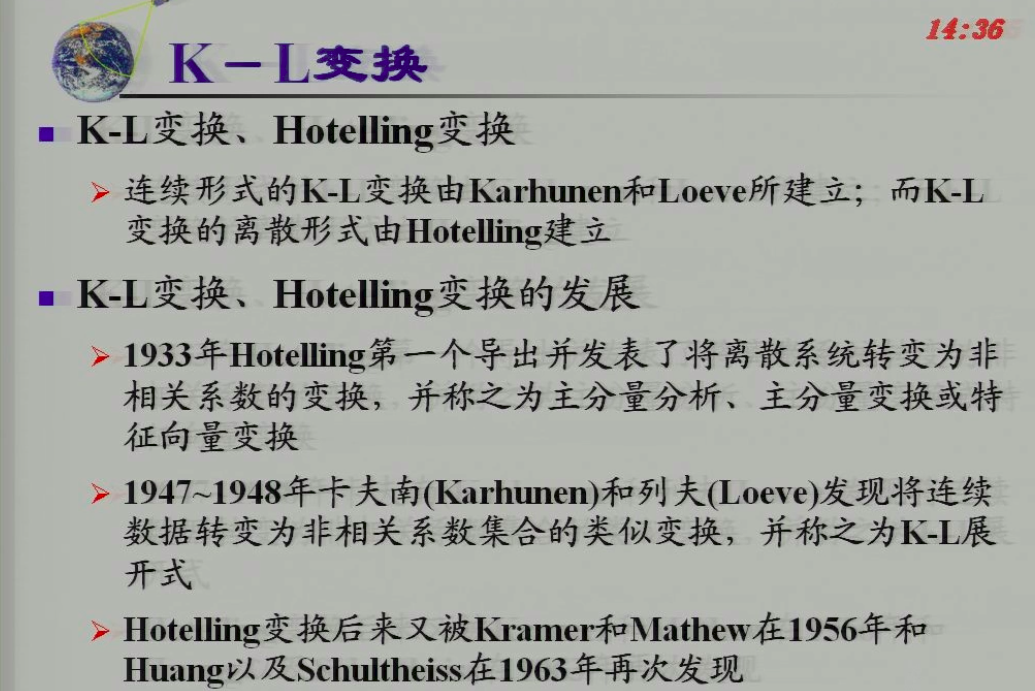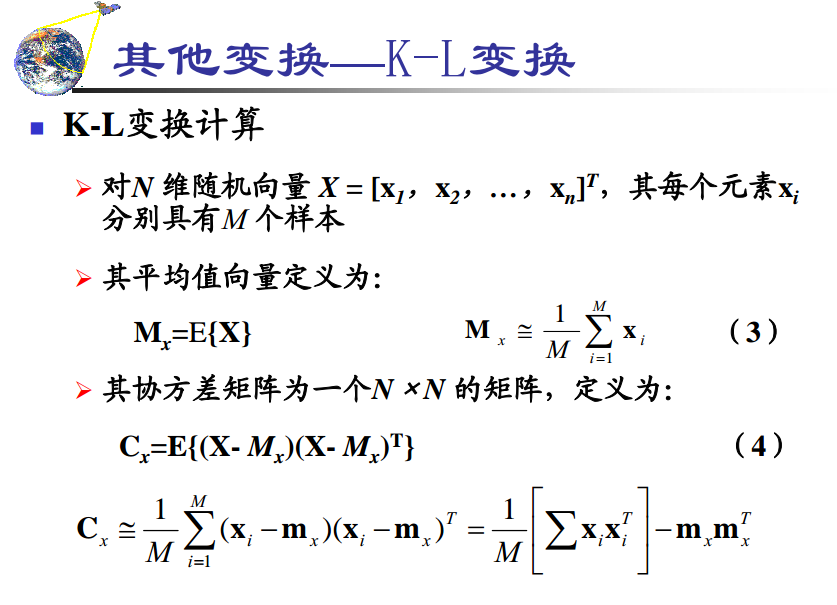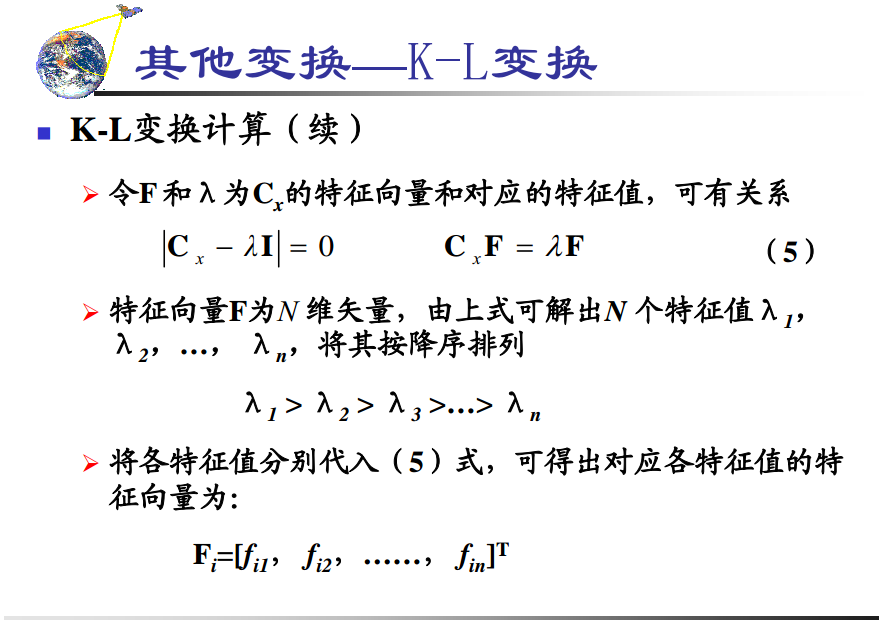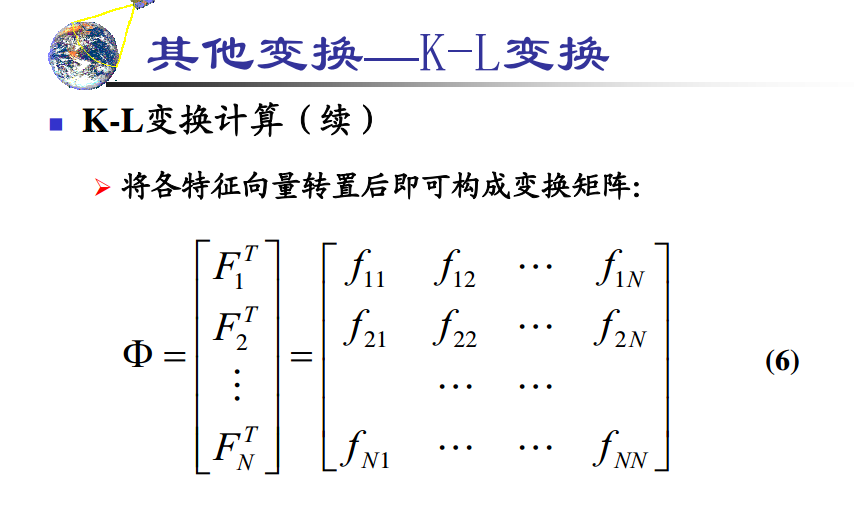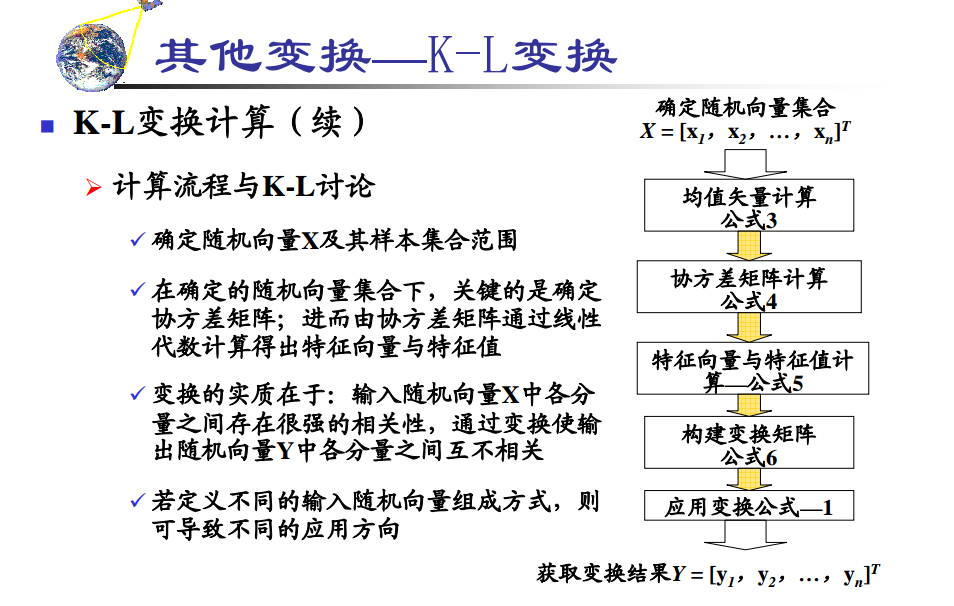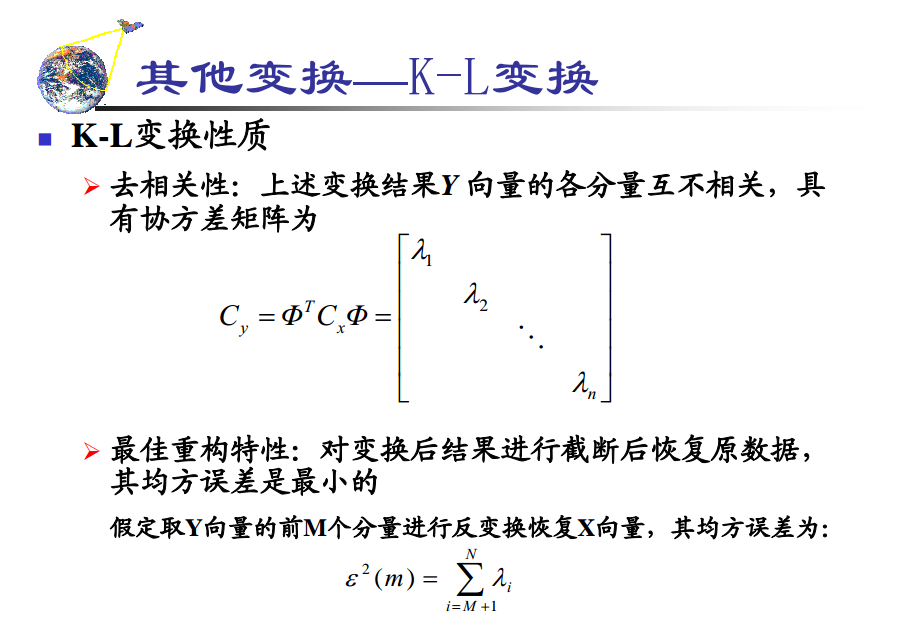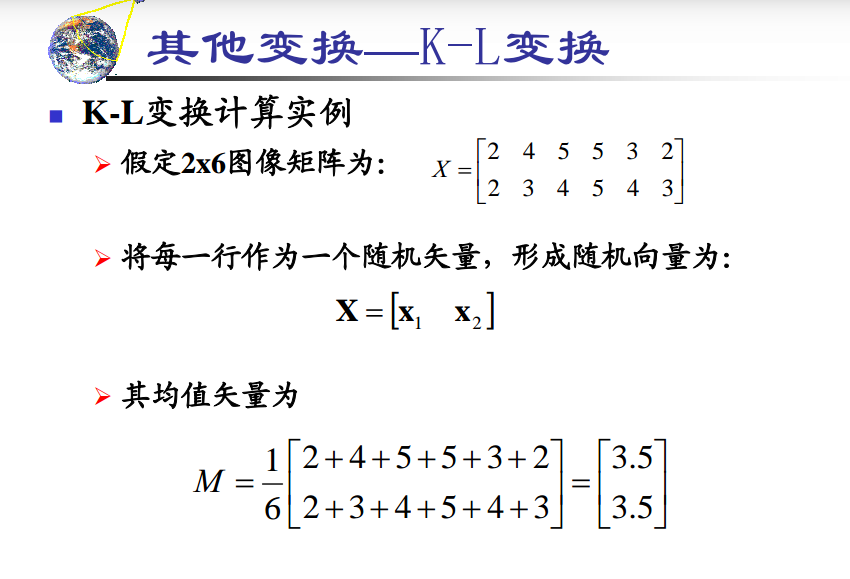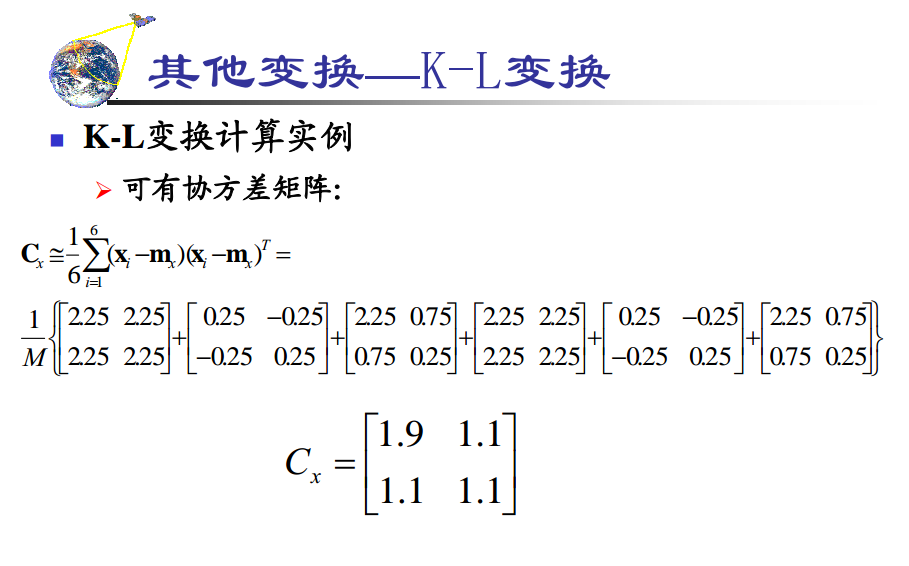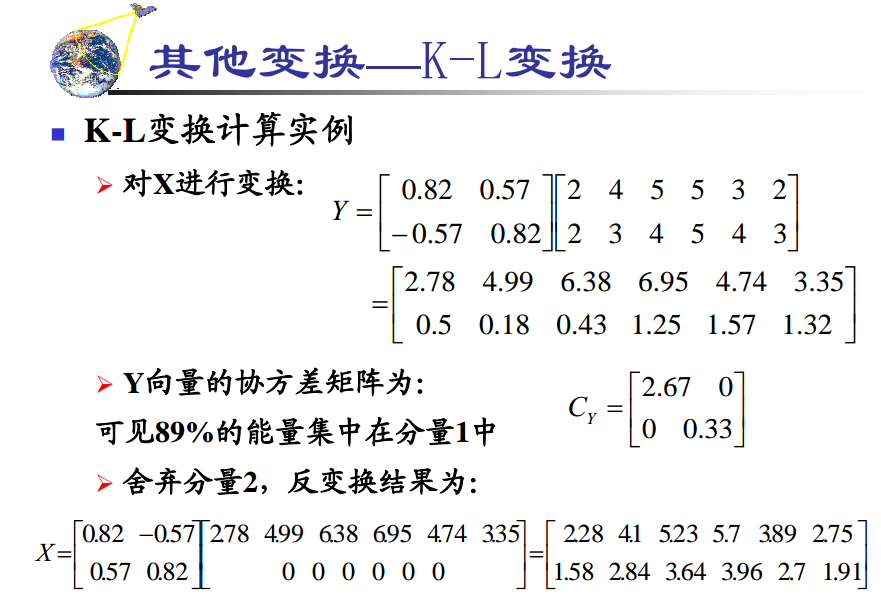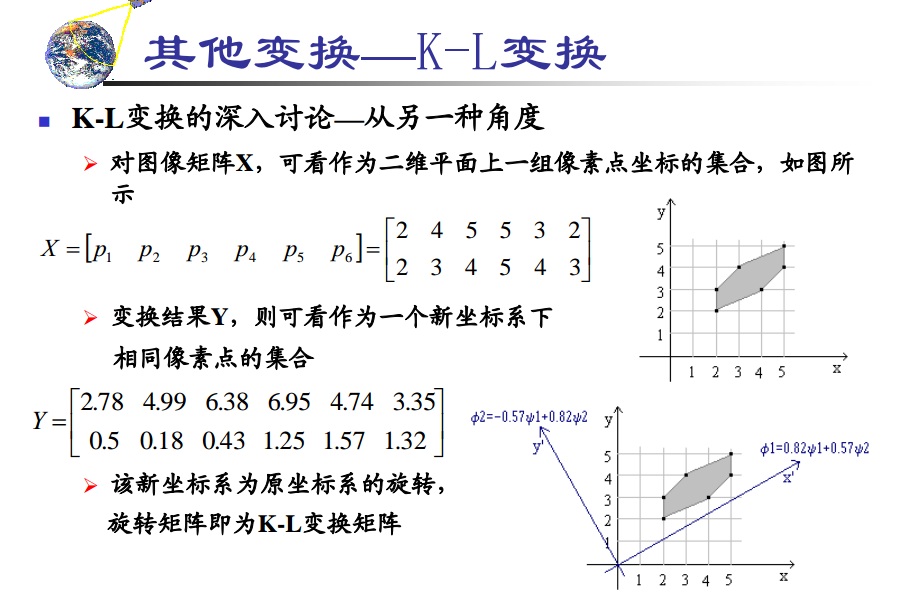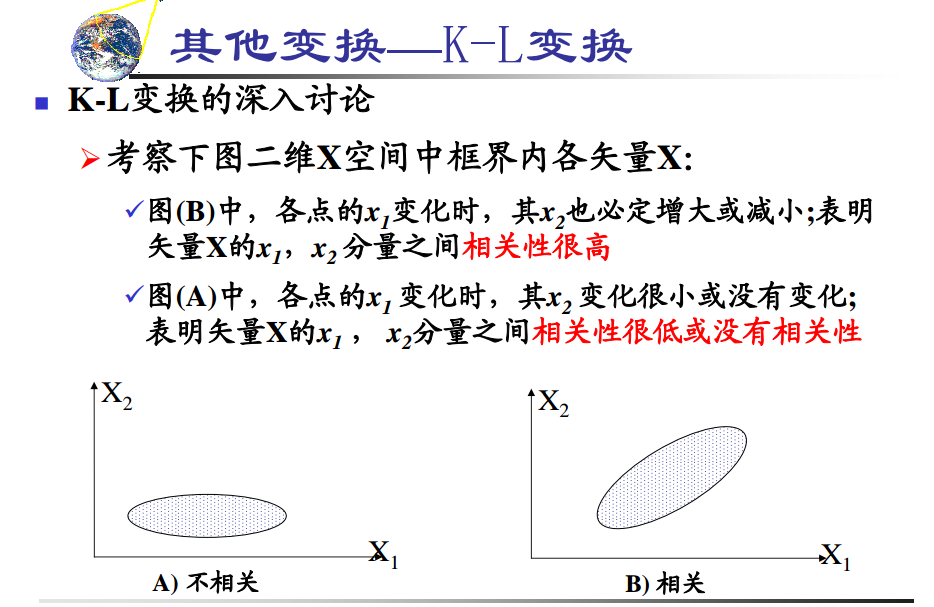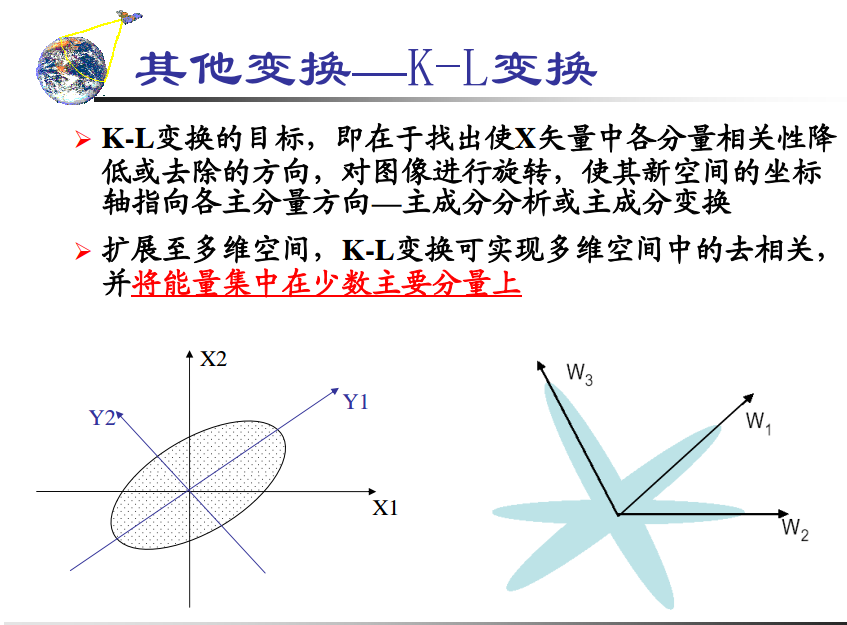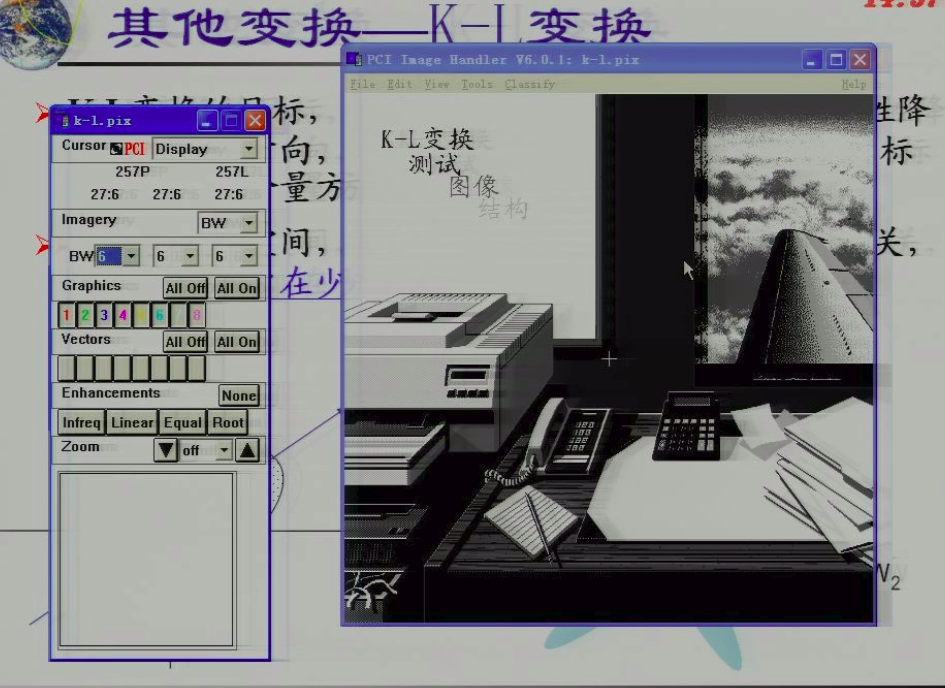### 另外一种比较基础的就是只是在一副图像中运用，使得图像旋转，指向图像的主分量方向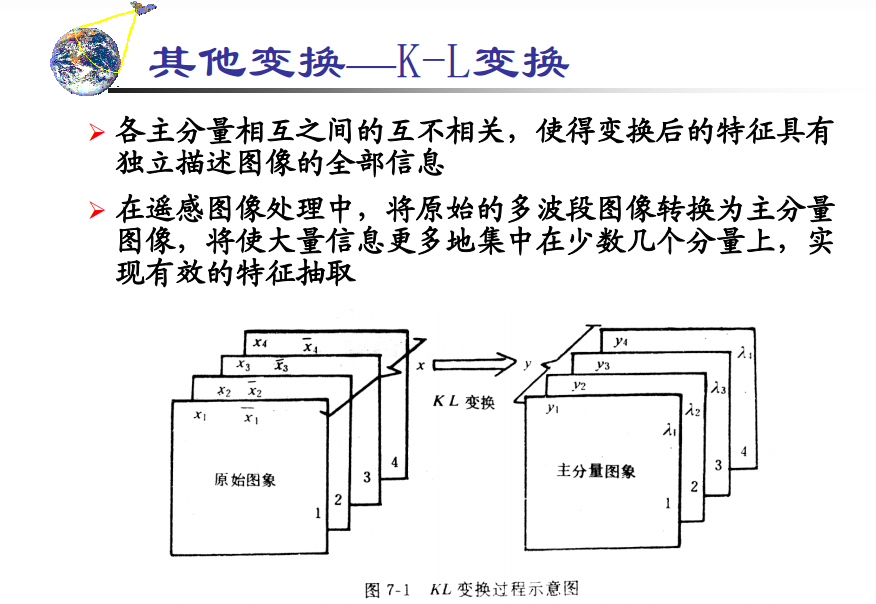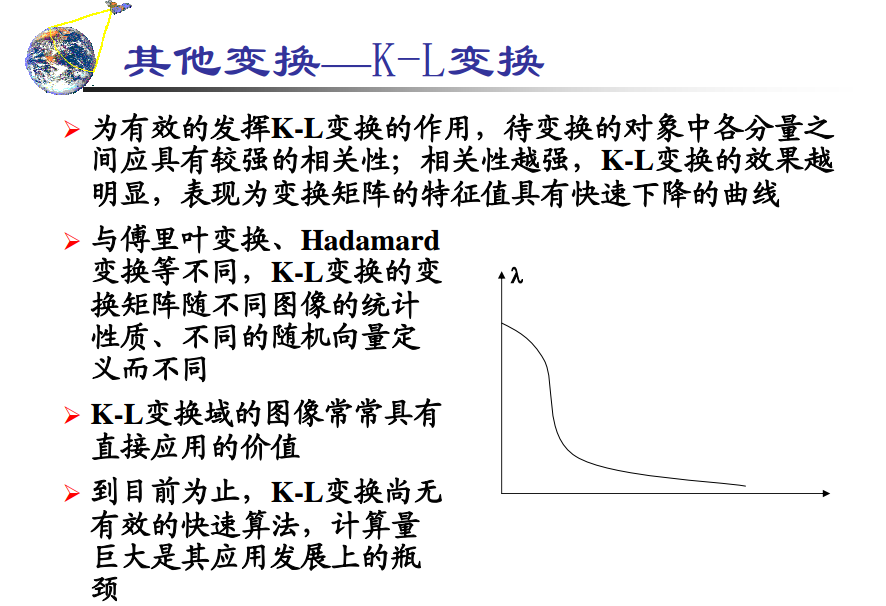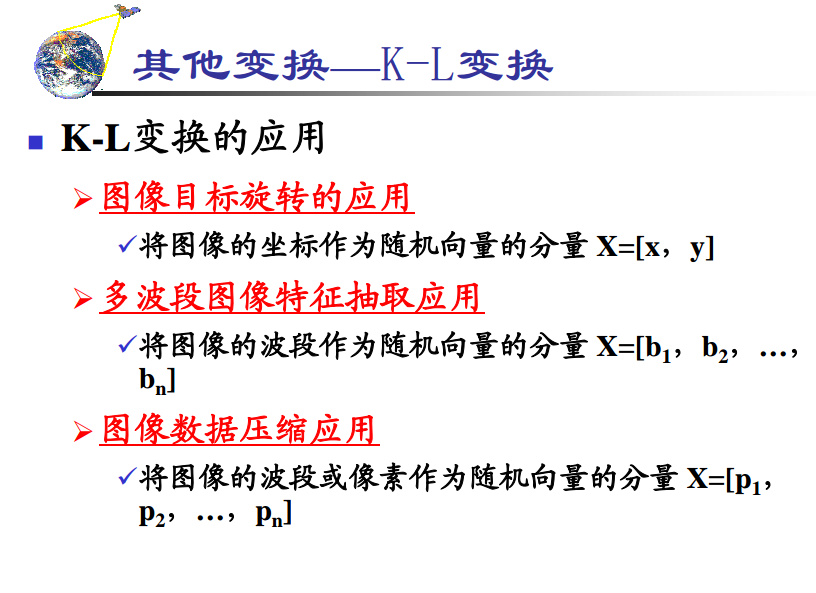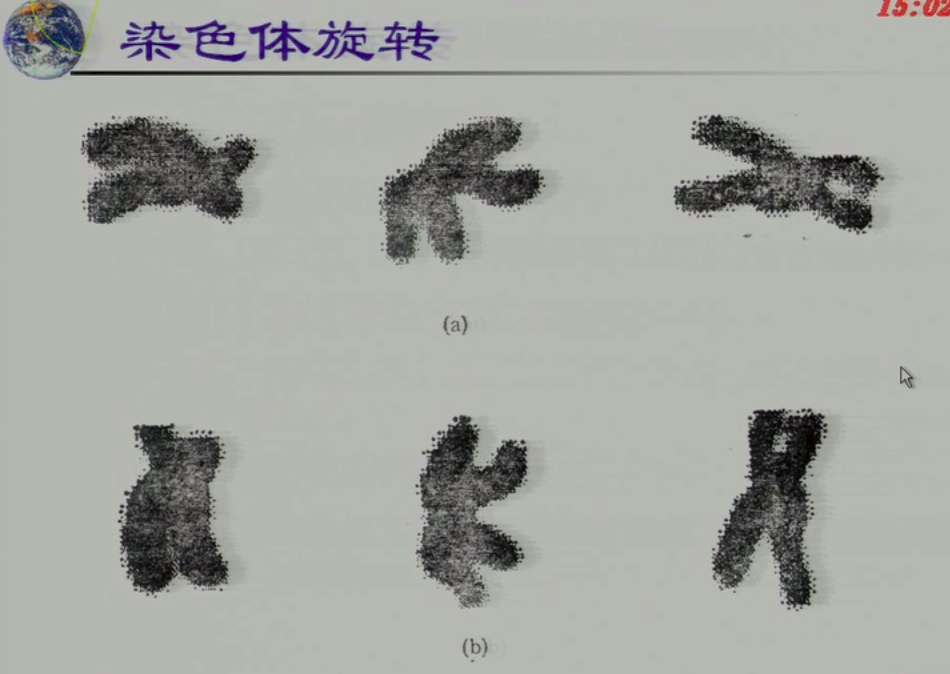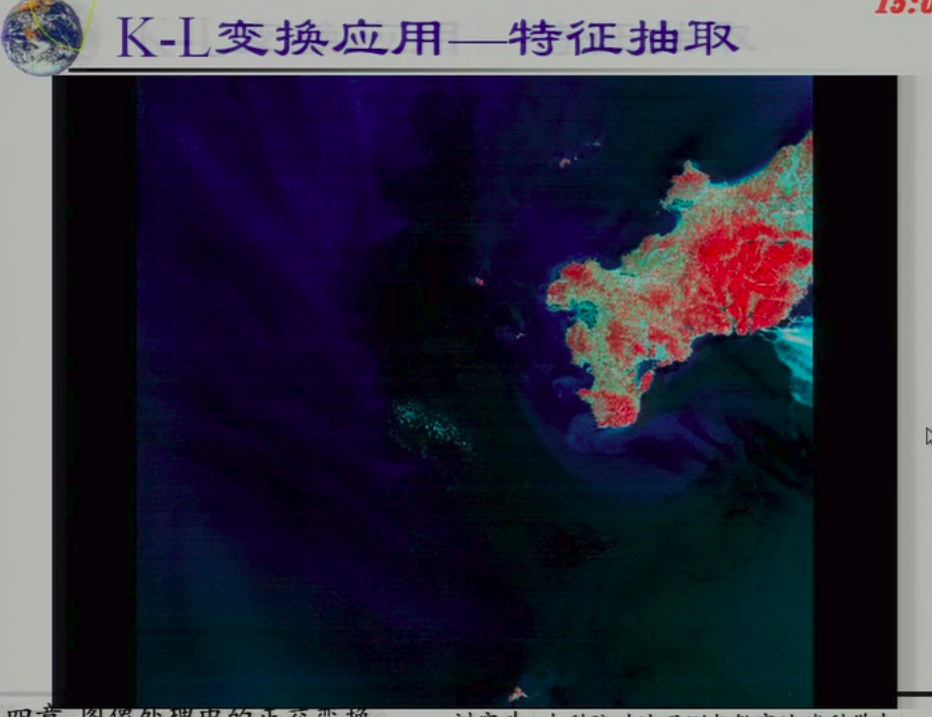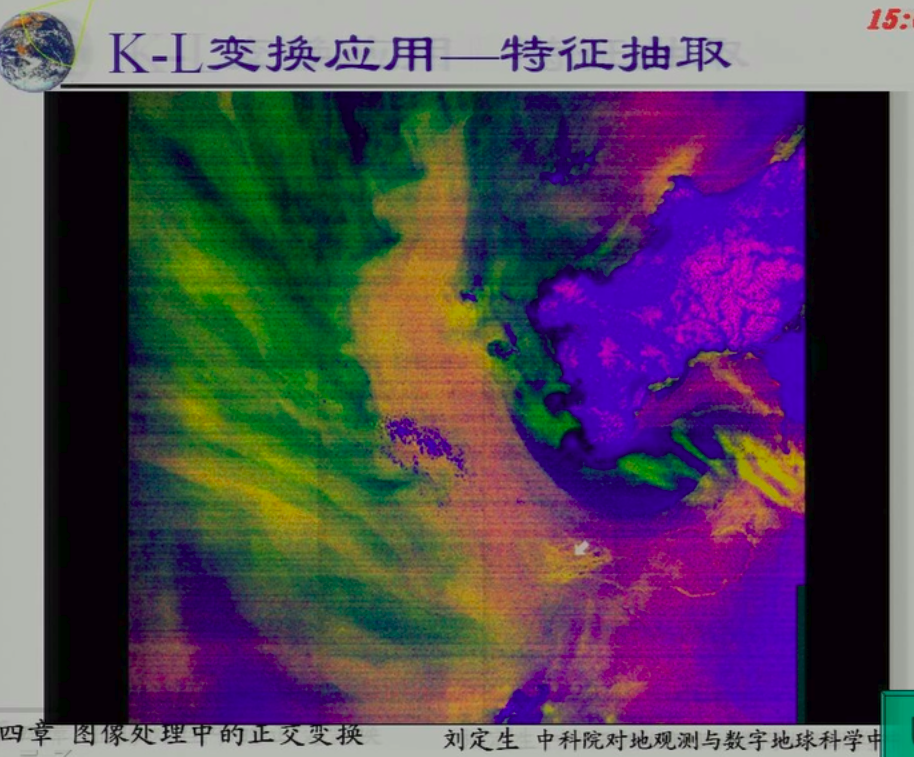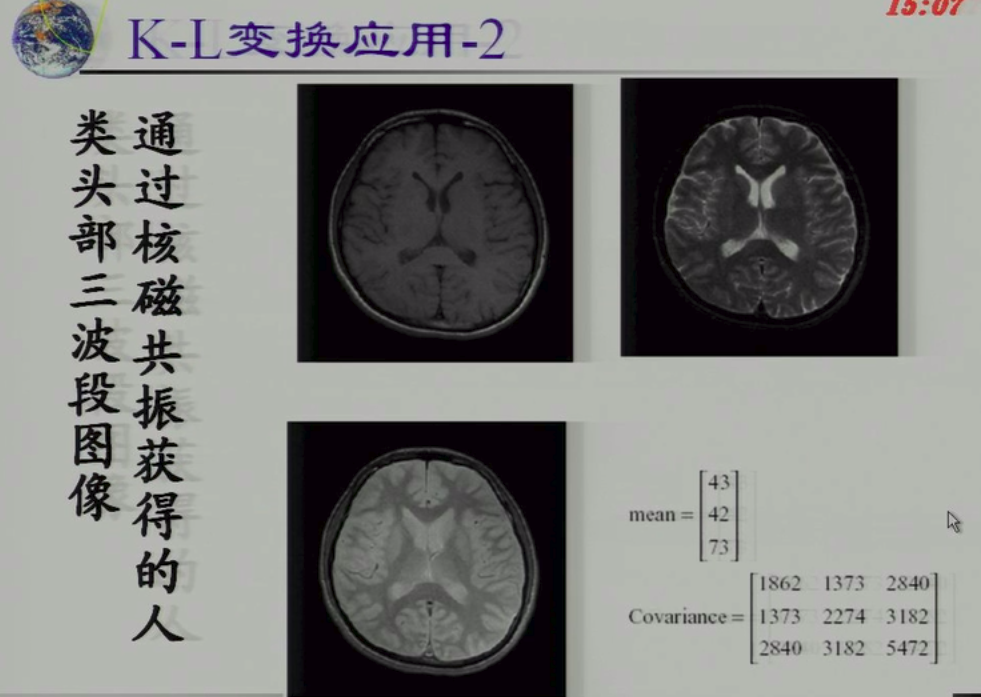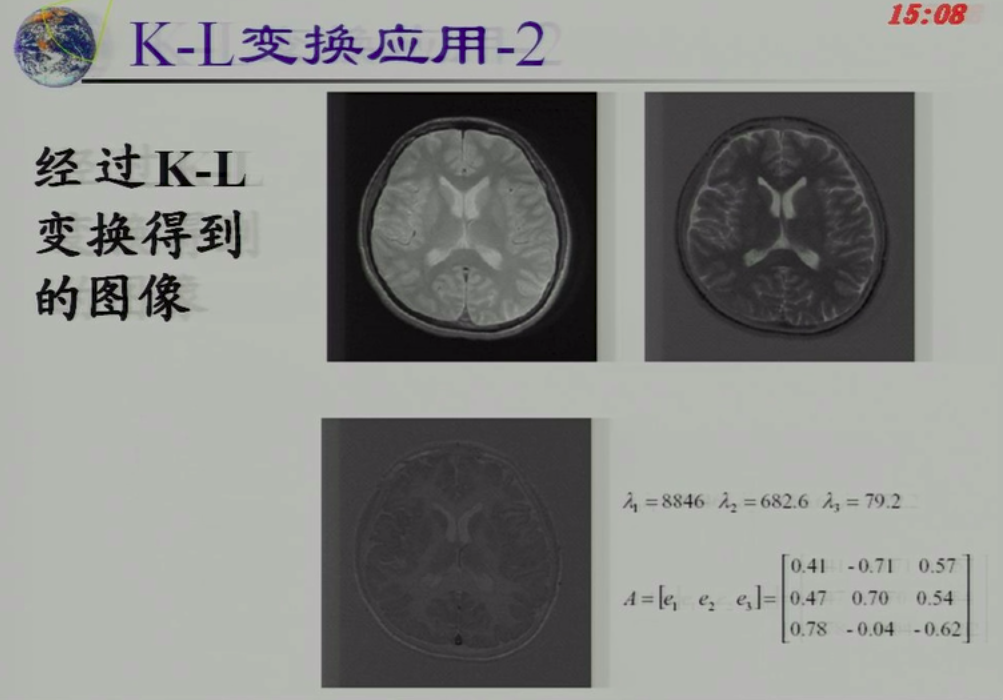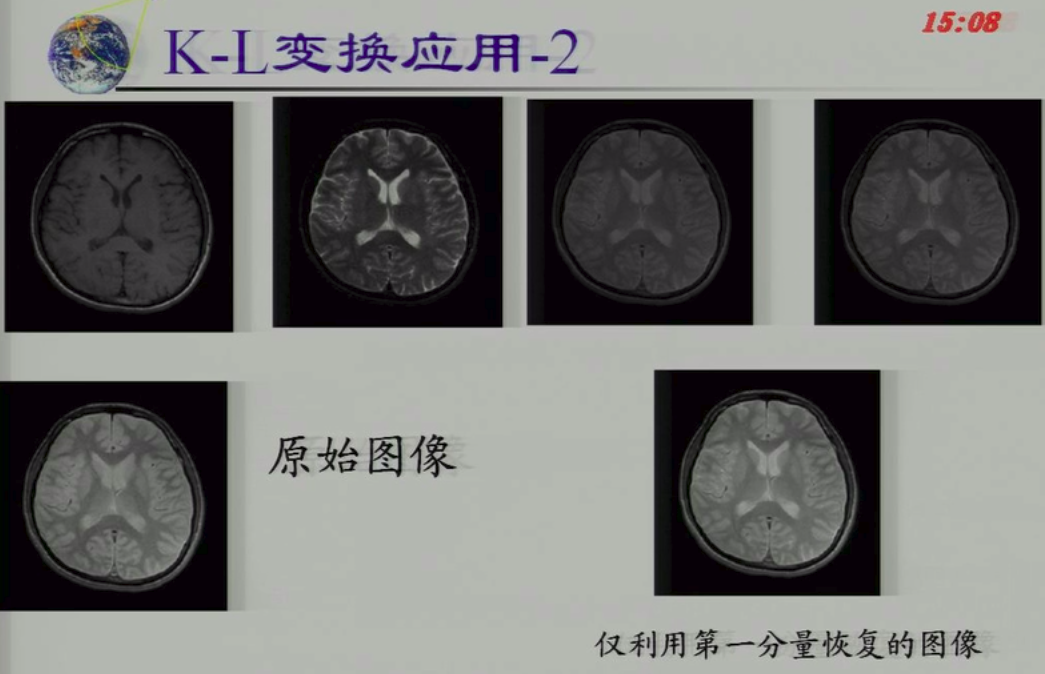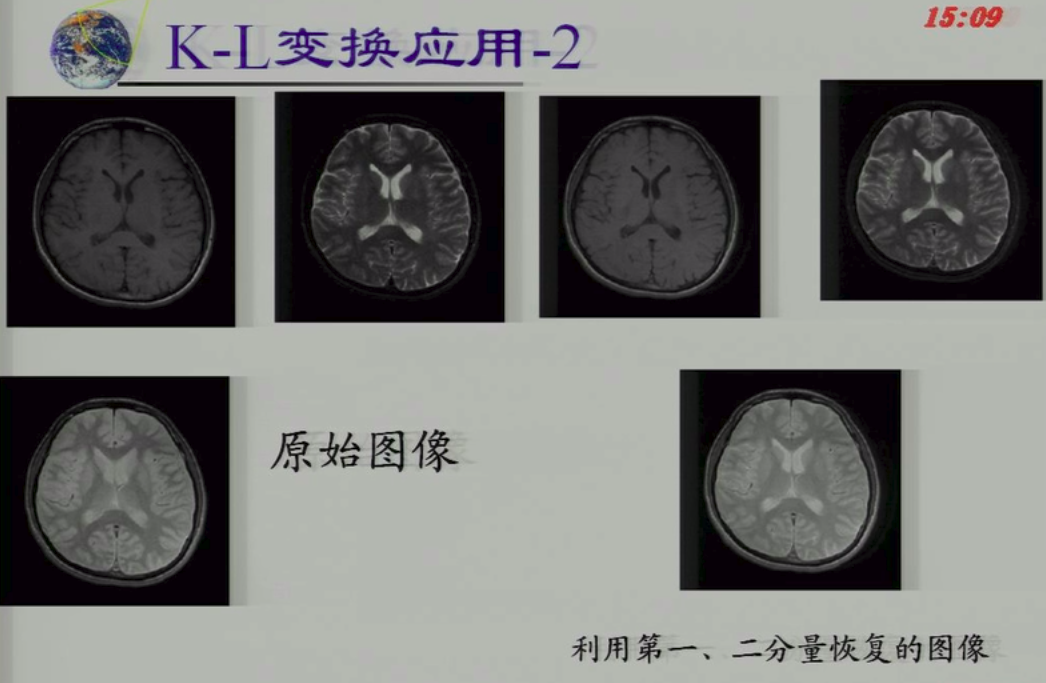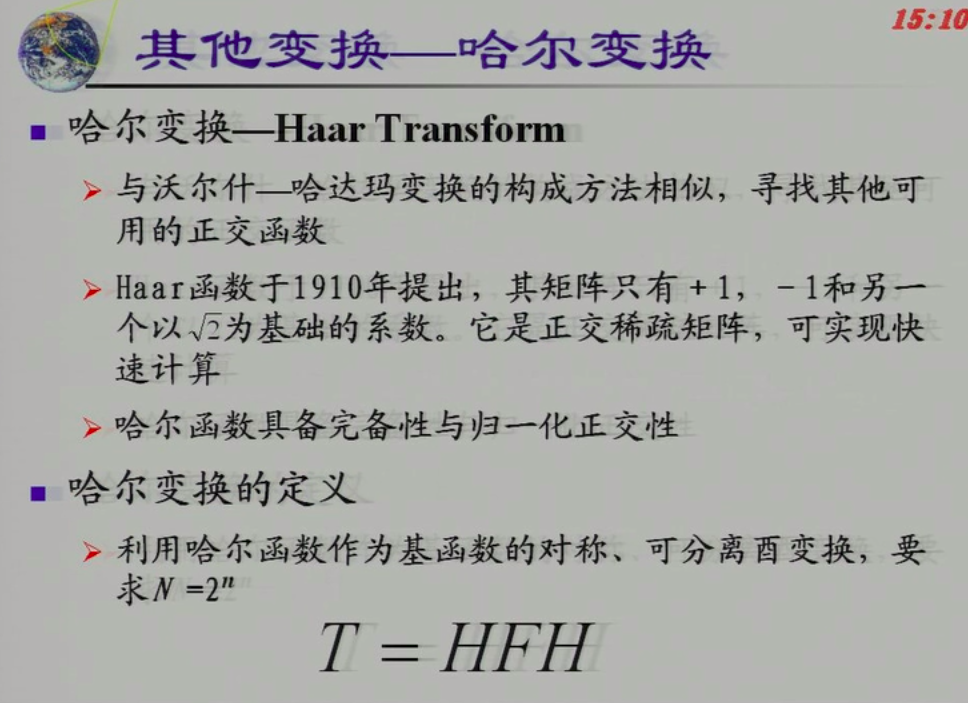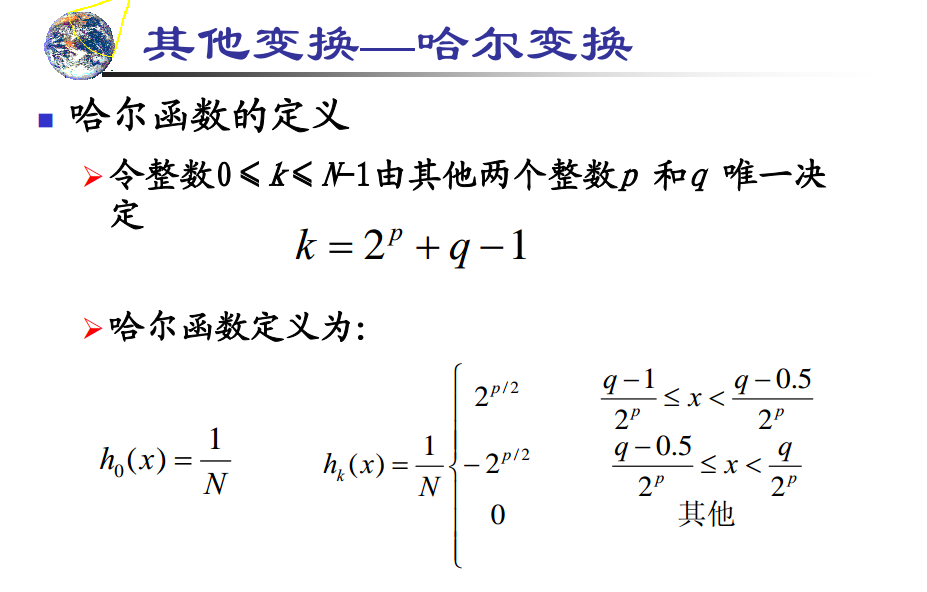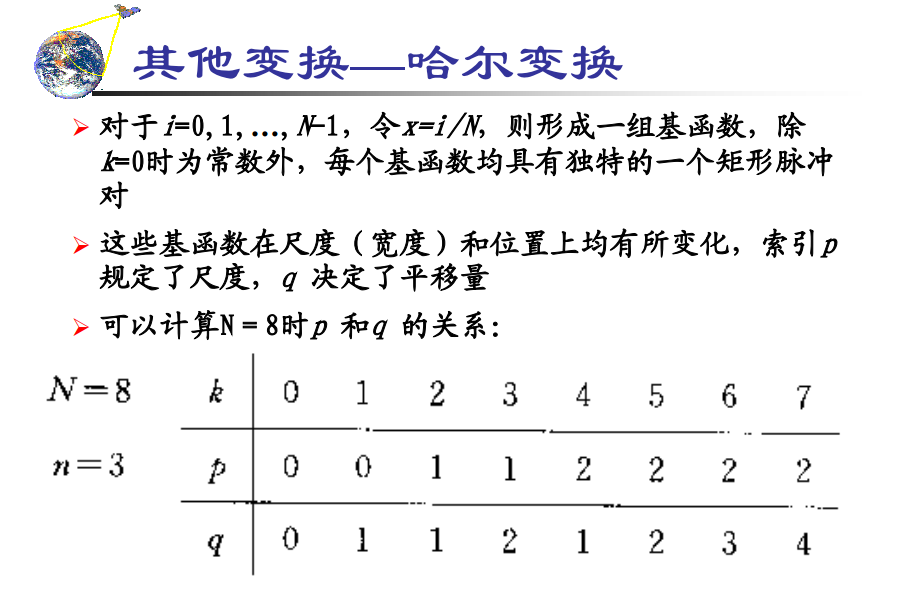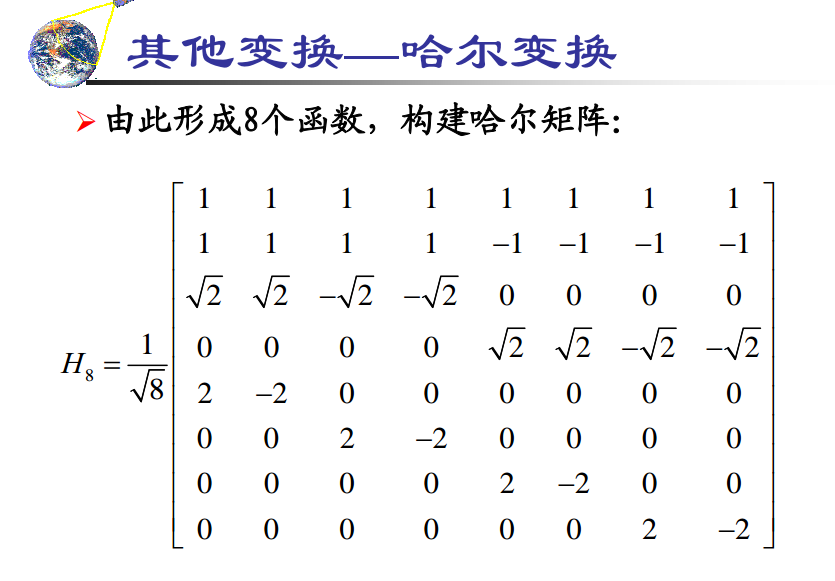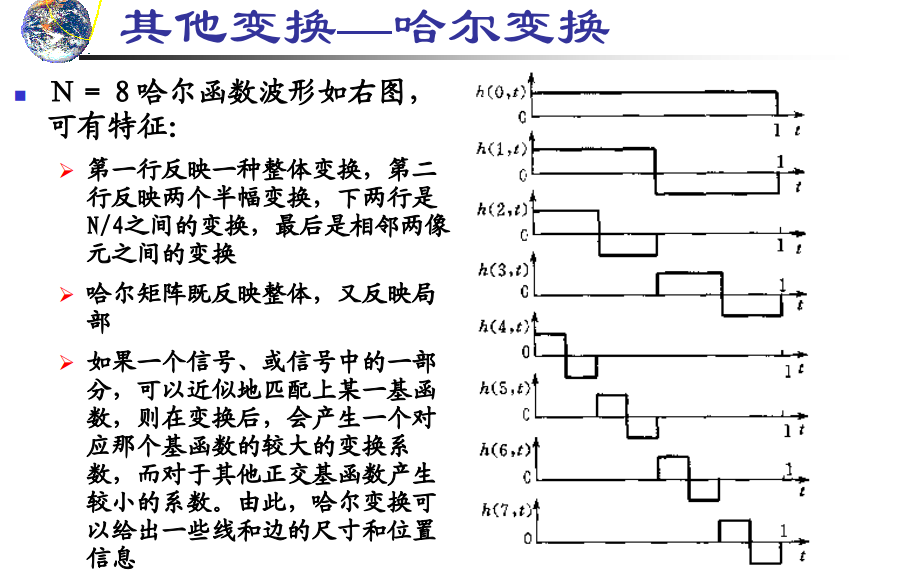### 所以哈尔矩阵既反映局部特征，又反映整体特征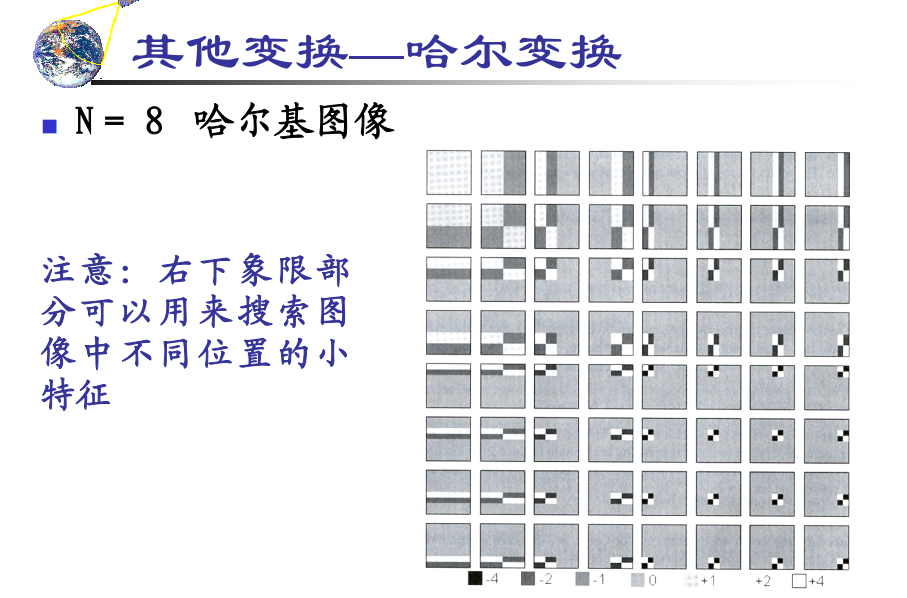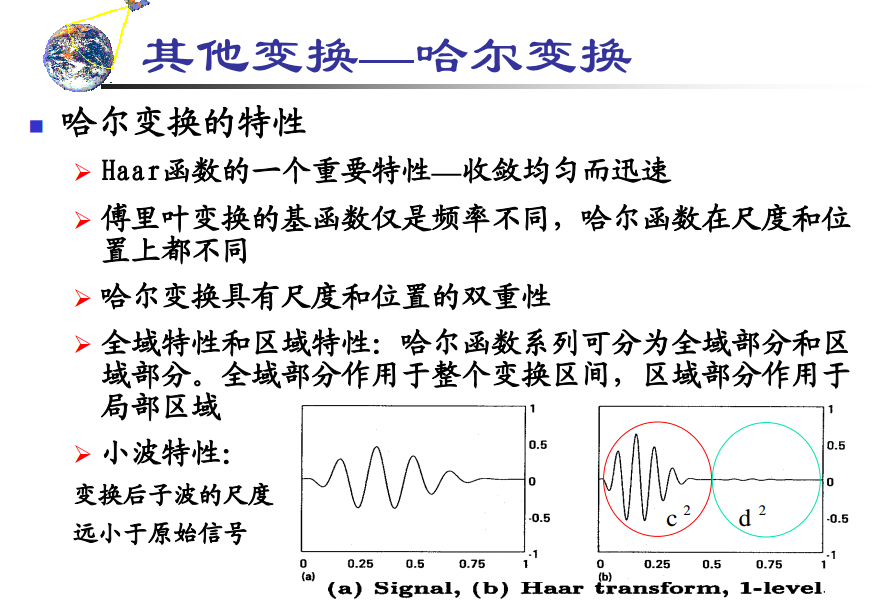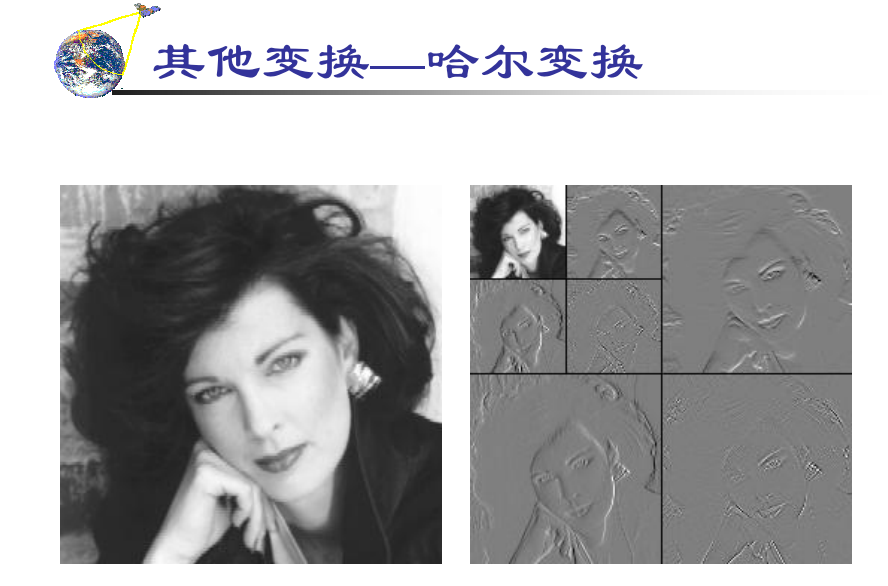2019-05-07 20:33:00 qq_36607894 阅读数 129

# KL最优变换推导

the Karhunen–Loève transform (KLT)，也叫the Karhunen–Loève expansion or Karhunen–Loève decomposition。

## 先进行无公式讲解（全是冗余）

KL变换也叫霍特林变换（Hotelling transform） ，特征向量变换（eigenvector transform）。它最初来源于随机过程领域，用于把一个随机过程表示成无穷多个正交函数的线性组合，即以无穷级数的形式表示随机过程，类似于用傅里叶级数表示一个有界区间上的函数。但区别是，傅里叶级数展开的系数是固定的（fixed）且基函数都是正弦型函数（即 either sin or cos），KL展开的系数是随机变量基函数依赖于过程本身，要通过过程本身来计算使用的基函数。

KLT是建立在统计特性基础上的变换，它是均方差(MSE, Mean Square Error)意义下的最佳变换，因此在压缩技术中占有重要地位。压缩就是把数据的分布尽量变得随机和不相关，以便用最少的空间去存储但又能正确表示，完全随机的数据比如白噪声就无法再压缩了。

PCA，主成分分析，在图像处理等信号处理领域用的很多，实际上就是离散KL变换，虽然PCA的变换矩阵是协方差阵C而KLT是相关矩阵R，但KLT变换前的数据是中心化了的（均值为0），C和R完全一样，所以PCA就是离散KL变换，一点区别都没有！

## 下面从最小化均方差的思路出发，手推出KLT（压缩后的干货）

n*n的正交变换矩阵$A^T=[\varphi_0,\varphi_1,\ldots,\varphi_{n-1}]$, \varphi_i^T\varphi_j=\left\{ \begin{aligned} & 1，i=j \\ & 0 ，i \not= j \\ \end{aligned} \right.

$y_i$是展开系数，$\varphi_i$是正交基函数，用这n个基函数的线性组合可以表示这个信源X。

$\hat{X}=\sum_{i=0}^{m-1}y_i\varphi_i$

$\epsilon=E[(X-\hat{X})^T(X-\hat{X})]=E[(\sum_{i=m}^{n-1}y_i\varphi_i)^T(\sum_{i=m}^{n-1}y_i\varphi_i)]=E[\sum_{i=m}^{n-1}y_i^2]=\sum_{i=m}^{n-1}E[y_i^2]=$
$\sum_{i=m}^{n-1}E[(y_i)(y_i)^T]=\sum_{i=m}^{n-1}E[(\varphi_i^TX)(\varphi_i^TX)^T]=\sum_{i=m}^{n-1}E[\varphi_i^TXX^T\varphi_i]=$
$\sum_{i=m}^{n-1}\varphi_i^TK_{XX}\varphi_i,\varphi_i^T\varphi_i=1.$

$min\quad \epsilon=\sum_{i=m}^{n-1}\varphi_i^TK_{XX}\varphi_i,\quad s.t. \quad \varphi_i^T\varphi_i-1=0.$

$L(x,\lambda)=\epsilon -\sum_{i=m}^{n-1}\lambda_i(\varphi_i^T\varphi_i-1)$

$min\quad L(x,\lambda)$

$\frac{\partial L(x,\lambda)}{\partial \varphi_i}=0$

$\frac{\partial [\sum_{i=m}^{n-1}\varphi_i^TK_{XX}\varphi_i-\sum_{i=m}^{n-1}\lambda_i(\varphi_i^T\varphi_i-1)]}{\partial \varphi_i}=0$

$\sum_{i=m}^{n-1}[2K_{XX}\varphi_i-2\lambda_i\varphi_i]=0$

$K_{XX}\varphi_i-\lambda_i\varphi_i=0$

$K_{XX}\varphi_i=\lambda_i\varphi_i$

so！！！

$K_{YY}=E[YY^T]=E[AXX^TA^T]=AK_{XX}A^T= \left[ \begin{matrix} \varphi_0^T \\ \varphi_1^T \\ \varphi_2^T \\ \vdots\\ \varphi_{n-1}^T \end{matrix} \right] K_{XX} \left[ \begin{matrix} \varphi_0 & \varphi_1 & \varphi_2 \cdots & \varphi_{n-1} \\ \end{matrix} \right]$
$\because K_{XX}\varphi_i=\lambda_i\varphi_i$

$\therefore [K_{XX}\varphi_i]^T=[\lambda_i\varphi_i]^T$

$\therefore \varphi_i^TK_{XX}=\lambda_i\varphi_i^T$

$\therefore K_{YY}= \left[ \begin{matrix} \lambda_0\varphi_0^T \\ \lambda_1 \varphi_1^T \\ \lambda_2 \varphi_2^T \\ \vdots\\ \lambda_{n-1} \varphi_{n-1}^T \end{matrix} \right] \left[ \begin{matrix} \varphi_0 & \varphi_1 & \varphi_2 \cdots & \varphi_{n-1} \\ \end{matrix} \right] = \left[ \begin{matrix} \lambda_0 & 0 & 0& \cdots & 0 \\ 0 & \lambda_1 & 0 &\cdots & 0 \\ \vdots & \vdots &\vdots &\ddots& \vdots & \\ 0 & 0 & 0& \cdots & \lambda_{n-1} \\ \end{matrix} \right]$

so！！！

$K_{YY}$是对角阵，说明Y的元素之间不再有相关性，去相关的目的达到了，压缩变换成功！